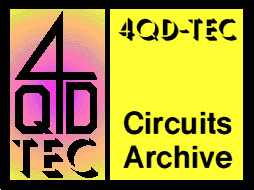# 4QD-TEC Electronics Circuits Reference Archive How Pulse Width Modulation works

## What is PWM and why is it useful?

Consider a waveform such as this: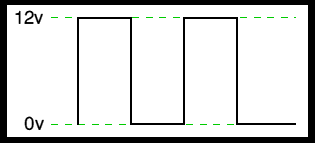it is a voltage switching between 0v and 12v. It is fairly obvious that, since the voltage is at 12v for exactly as long as it is at 0v, then a 'suitable device' connected to its output will see the average voltage and think it is being fed 6v - exactly half of 12v.

So by varying the width of the positive pulse - we can vary the 'average' voltage.

Similarly, if the switches keep the voltage at 12 for 3 times as long as at 0v, the average will be 3/4 of 12v - or 9v, as shown below.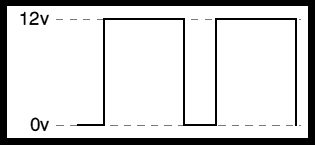and if the output pulse of 12v lasts only 25% of the overall time, then the average is 25% of 12v - or 3v.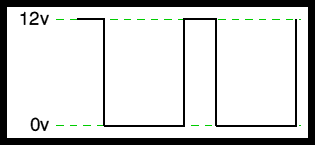By varying - or 'modulating' - the time that the output is at 12v (i.e. the width of the positive pulse) we can alter the average voltage. So we are doing 'pulse width modulation'.

I said earlier that the output had to feed 'a suitable device'. A radio would not work from this: the radio would see 12v then 0v, and would probably not work properly. However a device such as a motor will respond to the average, so PWM is a natural for motor control.

## A Pulse Width modulator

So, how do we generate a PWM waveform? It's actually very easy, there are circuits available in the TEC site. First you generate a triangle waveform as shown in the diagram below. You compare this with a d.c voltage, which you adjust to control the ratio of on to off time that you require. When the triangle is above the 'demand' voltage, the output goes high. When the triangle is below the demand voltage, the output goes low.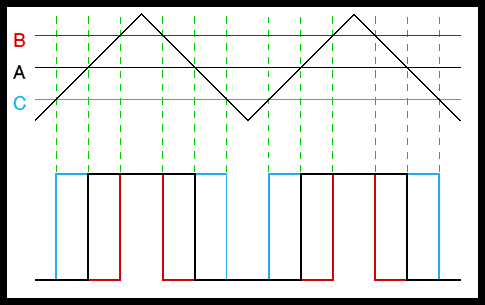When the demand speed it in the middle (A) you get a 50:50 output, as in black. Half the time the output is high and half the time it is low.

Fortunately, there is an IC (Integrated circuit) called a comparator: these come usually 4 sections in a single package. One can be used as the oscillator to produce the triangular waveform and another to do the comparing, so a complete oscillator and modulator can be done with half an IC and maybe 7 other bits.

The triangle waveform, which has approximately equal rise and fall slopes, is one of the commonest used, but you can use a sawtooth (where the voltage falls quickly and rinses slowly). You could use other waveforms and the exact linearity (how good the rise and fall are) is not too important.